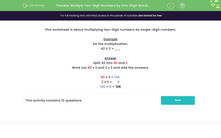# Multiply Two-Digit Numbers by One-Digit Numbers

In this worksheet, students will multiply two-digit numbers by one-digit numbers by splitting the larger number if necessary. They will use a formal written method.Key stage:  KS 2

Curriculum topic:   Number: Multiplication and Division

Curriculum subtopic:   Use Written Form for Multiplication

Difficulty level:#### Worksheet Overview

This activity is about multiplying two-digit numbers by single-digit numbers.

Example

Do the multiplication.

42 x 3 = __

Split 42 into 40 and 2

Work out 40 x 3 and 2 x 3 and add the answers.

40 x 3 = 120  (remember that 4 x 3 = 12 so 40 x 3 = 120)

2 x 3 =  6

120 +  6 = 126

Are you happy with that?Let's get started then!

### What is EdPlace?

We're your National Curriculum aligned online education content provider helping each child succeed in English, maths and science from year 1 to GCSE. With an EdPlace account you’ll be able to track and measure progress, helping each child achieve their best. We build confidence and attainment by personalising each child’s learning at a level that suits them.

Get started